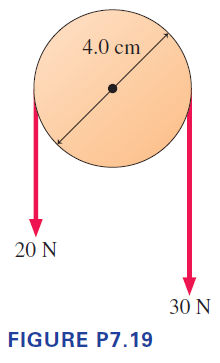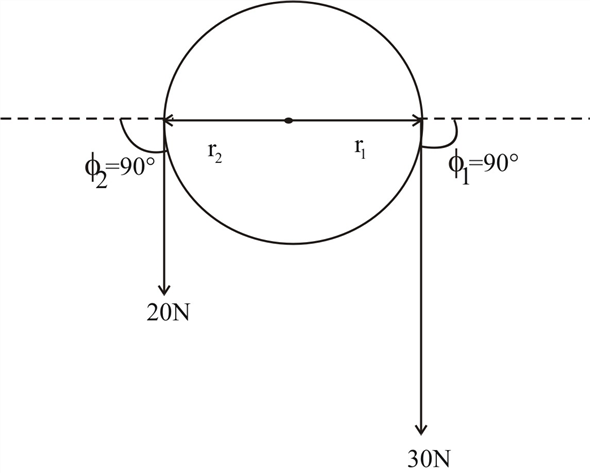Problem

What is the net torque about the axle on the pulley in Figure P7.19?...

What is the net torque about the axle on the pulley in Figure P7.19?Step-by-Step Solution

Solution 1

Diameter of the wheel = 4 cm

Radius of the wheel r = 2 cm = 0.02 mTorque is the rotational equivalent of force

$$\tau=r F \sin \phi$$

Net torque on the pulley about the axle is the sum of the torque due to the $$30 \mathrm{~N}$$ force and the torque due to the $$20 \mathrm{~N}$$ force.

\begin{aligned} &\tau=(30 \mathrm{~N}) r_{1} \sin \phi_{1}+(20 \mathrm{~N}) r_{2} \sin \phi_{2} \\ &=(30 \mathrm{~N})(0.02 \mathrm{~m}) \sin \left(-90^{\circ}\right)+(20 \mathrm{~N})(0.02 \mathrm{~m}) \sin 90^{\circ} \\ &=(-0.6 \mathrm{Nm})+(0.4 \mathrm{Nm}) \\ &=-0.2 \mathrm{Nm} \end{aligned}

The net torque is negative, so the pulley moves in a clockwise motion.